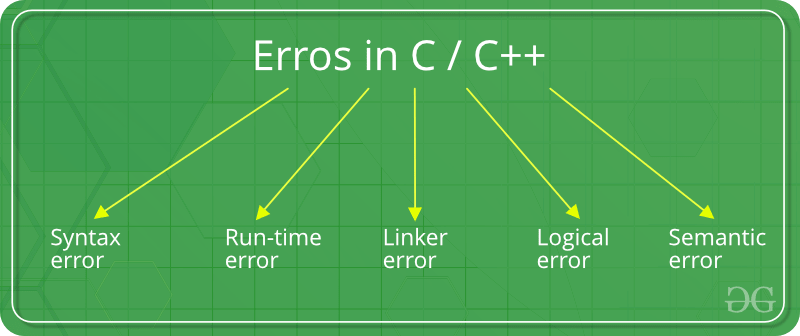Open In App

# Errors in C/C++

Error is an illegal operation performed by the user which results in abnormal working of the program.
Programming errors often remain undetected until the program is compiled or executed. Some of the errors inhibit the program from getting compiled or executed. Thus errors should be removed before compiling and executing.
The most common errors can be broadly classified as follows.

Type of errors:1. Syntax errors: Errors that occur when you violate the rules of writing C/C++ syntax are known as syntax errors. This compiler error indicates something that must be fixed before the code can be compiled. All these errors are detected by compiler and thus are known as compile-time errors.
Most frequent syntax errors are:
• Missing Parenthesis (})
• Printing the value of variable without declaring it
• Missing semicolon like this:

## C++

 `// C++ program to illustrate``// syntax error` `#include ``using` `namespace` `std;` `void` `main()``{``    ``int` `x = 10;``    ``int` `y = 15;``    ` `    ``cout << ``" "``<< (x, y) ``// semicolon missed``}` `// This code is contributed by shivanisinghss2110`

## C

 `// C program to illustrate``// syntax error``#include``void` `main()``{``    ``int` `x = 10;``    ``int` `y = 15;``    ` `    ``printf``(``"%d"``, (x, y)) ``// semicolon missed``}`

Error:

`error: expected ';' before '}' token`
• Syntax of a basic construct is written wrong. For example : while loop

## C++

 `// C++ program to illustrate``// syntax error``#include ``using` `namespace` `std;` `int` `main(``void``)``{``    ``// while() cannot contain "." as an argument.``    ``while``(.)``    ``{``        ``cout <<``"hello"``;``    ``}``    ``return` `0;``}` `// This code is contributed by shivanisinghss2110`

## C

 `// C program to illustrate``// syntax error``#include``int` `main(``void``)``{``    ``// while() cannot contain "." as an argument.``    ``while``(.)``    ``{``        ``printf``(``"hello"``);``    ``}``    ``return` `0;``}`

Error:

```error: expected expression before '.' token
while(.) ```
• In the given example, the syntax of while loop is incorrect. This causes a syntax error.
1. Run-time Errors : Errors which occur during program execution(run-time) after successful compilation are called run-time errors. One of the most common run-time error is division by zero also known as Division error. These types of error are hard to find as the compiler doesn’t point to the line at which the error occurs.
For more understanding run the example given below.

## C++

 `// C++ program to illustrate``// run-time error``#include ``#include ``using` `namespace` `std;` `void` `main()``{``    ``int` `n = 9, ``div` `= 0;``  ` `    ``// wrong logic``    ``// number is divided by 0,``    ``// so this program abnormally terminates``    ``div` `= n/0;``    ` `   ``cout << ``"result = "``<< ``div``;``}` `// This code is contributed by shivanisinghss2110`

## C

 `// C program to illustrate``// run-time error``#include``void` `main()``{``    ``int` `n = 9, ``div` `= 0;``  ` `    ``// wrong logic``    ``// number is divided by 0,``    ``// so this program abnormally terminates``    ``div` `= n/0;``    ` `    ``printf``(``"result = %d"``, ``div``);``}`

Error:

```warning: division by zero [-Wdiv-by-zero]
div = n/0;```
1. In the given example, there is Division by zero error. This is an example of run-time error i.e errors occurring while running the program.
2. Linker Errors: These error occurs when after compilation we link the different object files with main’s object using Ctrl+F9 key(RUN). These are errors generated when the executable of the program cannot be generated. This may be due to wrong function prototyping, incorrect header files. One of the most common linker error is writing Main() instead of main()

## C++

 `// C++ program to illustrate``// linker error``#include ``using` `namespace` `std;` `void` `Main() ``// Here Main() should be main()``{``    ``int` `a = 10;``    ``cout << ``" "``<< a;``}` `// This code is contributed by shivanisinghss2110`

## C

 `// C program to illustrate``// linker error``#include` `void` `Main() ``// Here Main() should be main()``{``    ``int` `a = 10;``    ``printf``(``"%d"``, a);``}`

Error:

`(.text+0x20): undefined reference to `main'`
1. Logical Errors : On compilation and execution of a program, desired output is not obtained when certain input values are given. These types of errors which provide incorrect output but appears to be error free are called logical errors. These are one of the most common errors done by beginners of programming.
These errors solely depend on the logical thinking of the programmer and are easy to detect if we follow the line of execution and determine why the program takes that path of execution.

## C++

 `// C++ program to illustrate``// logical error``int` `main()``{``    ``int` `i = 0;` `    ``// logical error : a semicolon after loop``    ``for``(i = 0; i < 3; i++);``    ``{``       ``cout << ``"loop "``;``        ``continue``;``    ``}``    ``return` `0;``}`  `// This code is contributed by shivanisinghss2110.`

## C

 `// C program to illustrate``// logical error``int` `main()``{``    ``int` `i = 0;` `    ``// logical error : a semicolon after loop``    ``for``(i = 0; i < 3; i++);``    ``{``        ``printf``(``"loop "``);``        ``continue``;``    ``}``    ``getchar``();``    ``return` `0;``}`

1. No output
2. Semantic errors : This error occurs when the statements written in the program are not meaningful to the compiler.

## C++

 `// C++ program to illustrate``// semantic error``int` `main()``{``    ``int` `a, b, c;``    ``a + b = c; ``//semantic error``}` `// This code is contributed by sarajadhav12052009`

## C

 `// C program to illustrate``// semantic error``void` `main()``{``  ``int` `a, b, c;``  ``a + b = c; ``//semantic error``}`

Error:

``` error: lvalue required as left operand of assignment
a + b = c; //semantic error```
This article is contributed by Krishna Bhatia. If you like GeeksforGeeks and would like to contribute, you can also write an article using write.geeksforgeeks.org or mail your article to review-team@geeksforgeeks.org. See your article appearing on the GeeksforGeeks main page and help other Geeks.